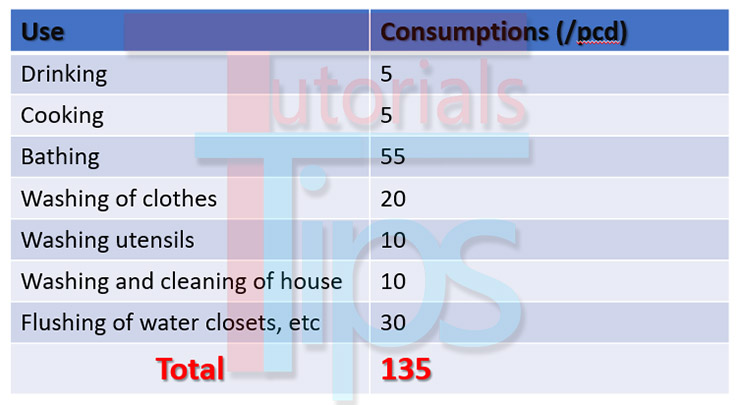# WATER DEMAND AND WATER TANK SIZE & VOLUME CALCULATION

### Domestic Water Demand

• The total domestic water consumption usually amounts to 50 to 60% of the total water consumption.
• The I.S. Code lays down a limit of water consumption between 135 to 225 litre per capita per day (/pcd).
• Under ordinary condition (as per I.S. code) the minimum domestic water demand for a town or a city with full flushing system Should be taken at 200 lpcd. Although it can be reduced to 135 lpcd  for economical weaker section and LIG colonies depending upon prevailing conditions.

1. Minimum domestic water consumption (Annual average) for Indian town and cities with Full Flushing  systems as per 1172-1993.2. Minimum domestic water consumption (Annual average) for weaker section and LIG (Lower Income Group) Colonies in small Indian Towns and Cities.#### WATER TANK CAPACITY CALCULATION

For a Small  LIG (Lower Income Group) family  = 5 Members

Water Consumption in 1 Day  = 135 litre

For 5 member in a Day  = 135 x 5

For 5 member in a Day  = 675 lit.

Assume the water consumption 1000 lit.

We know water density is 1000 lit/m3

1m3 = 1000 lit.

Water tank volume is  = 1m3

#### WATER TANK SIZE CALCULATION

Water tank volume is  = 1m3

Volume of water tank is DxBxL

Assume water tank depth as your required  = 0.5m

Depth is water tank is,  D= 0.5 m

Area of water tank  = 1/0.5 = 2

Area of water tank,   L x B  = 2 meter squre

Suppose L= 2B

2B X B = 2

B^2   =  2/2

B^2 =1

B = √1

B = 1

Width of water tank is, B= 1 m

Put value of B in assume equation,    Suppose L= 2B

L = 2X1

L = 2

Length of water tank is, L = 2 m

Overall water tank site is DxBxL

0.5x1x2 = 1 meter cube

Here is a plan of water tank and cross section of water tank

Excel Sheet For Size Calculation –